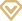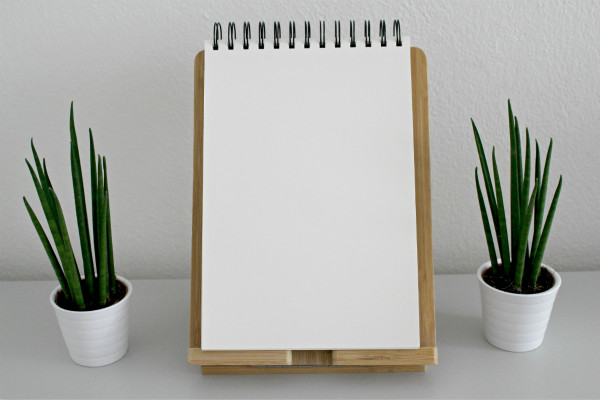# 小升初数学必备知识点汇总

2018-11-28 11:33:00   无忧考网     [ 字体： ] [文档预览 ] [文档下载 ]1.等式：等号左边的数值与等号右边的数值相等的式子叫做等式。等式的基本性质：等式两边同时乘以(或除以)一个相同的数，等式仍然成立。

2.方程式：含有未知数的等式叫方程式。

3.一元一次方程式：含有一个未知数，并且未知数的次数是一次的等式叫做一元一次方程式。学会一元一次方程式的例法及计算。即例出代有χ的算式并计算。

4.代数： 代数就是用字母代替数。

5.代数式：用字母表示的式子叫做代数式。

1.三角形的面积=底×高÷2。 公式 S= a×h÷2

2.正方形的面积=边长×边长 公式 S= a2

3.长方形的面积=长×宽 公式 S= a×b

4.平行四边形的面积=底×高 公式 S= a×h

5.梯形的面积=(上底+下底)×高÷2 公式 S=(a+b)h÷2

6.内角和：三角形的内角和=180度。

7.长方体的表面积=(长×宽+长×高+宽×高 ) ×2 公式：S=(a×b+a×c+b×c)×2

8.正方体的表面积=棱长×棱长×6 公式： S=6a2

9.长方体的体积=长×宽×高 公式：V = abh

10.长方体(或正方体)的体积=底面积×高 公式：V = abh

11.正方体的体积=棱长×棱长×棱长 公式：V = a3

12.圆的周长=直径×π 公式：L=πd=2πr

13.圆的面积=半径×半径×π 公式：S=πr2

14.圆柱的表(侧)面积：圆柱的表(侧)面积等于底面的周长乘高。公式：S=ch=πdh=2πrh

15.圆柱的表面积：圆柱的表面积等于底面的周长乘高再加上两头的圆的面积。公式：S=ch+2s=ch+2πr2

16.圆柱的体积：圆柱的体积等于底面积乘高。公式：V=Sh

17.圆锥的体积=1/3底面×积高。公式：V=1/3Sh

1.长度单位换算

1千米=1000米 1米=10分米 1分米=10厘米 1米=100厘米 1厘米=10毫米

2.面积单位换算

1平方千米=100公顷 1公顷=10000平方米 1平方米=100平方分米 1平方分米=100平方厘米 1平方厘米=100平方毫米

3.体(容)积单位换算

1立方米=1000立方分米 1立方分米=1000立方厘米 1立方分米=1升 1立方厘米=1毫升 1立方米=1000升

4.重量单位换算

1吨=1000 千克 1千克=1000克 1千克=1公斤

5.时间单位换算

1世纪=100年 1年=12月

1日=24小时 1时=60分 1分=60秒 1时=3600秒

1.平均数: 总数÷总份数＝平均数

2.和差问题：(和＋差)÷2＝大数 (和－差)÷2＝小数

3.和倍问题：和÷(倍数－1)＝小数

4.差倍问题：差÷(倍数－1)＝小数 小数×倍数＝大数 (或 小数＋差＝大数)

5.相遇问题

6.追及问题

7.流水问题

8.浓度问题

9.利润与折扣问题

10、盈亏问题

(盈＋亏)÷两次分配量之差＝参加分配的份数 (大盈－小盈)÷两次分配量之差＝参加分配　　的份数 (大亏－小亏)÷两次分配量之差＝参加分配的份数

1.圆周率常取数据

3.14×1＝3.14

3.14×2＝6.28

3.14×3＝9.42

3.14×4＝12.56

3.14×5＝15.7

3.15×6＝18.84

3.14×7＝21.98

3.14×8＝25.12

3.14×9＝28.26

2.常用特殊数的乘积

25×3＝75

25×4＝100

25×8＝200

125×3＝375

125×4＝500

125×8＝1000

625×16＝10000

37×3=111

3.常用平方数

112=121 122=144 132=169 142=196

152=225 162=256 172=289 182=324

192=361 102=100 202=400 302=900

402=1600 502=2500 602=3600 7702=4900

802=6400 152=225 252=625 352=1225

452=2025 552=3025 652=4225 752=5625

852=7225

4.关于常用分数与小数的互化

1/2=0.5 4=0.25 3/4=0.75 1/5=0.2 2/5=0.4

3/5=0.6 4/5=0.8 1/8=0.125 3/8=0.375 5/8=0.625

7/8=0.875 1/20=0.05 3/20=0.15 7/20=0.35

9/20=0.45 11/20=0.55 1/25=0.04 2/25=0.08

3/25=0.12 4/25=0.16 6/25=0.24

5.常用立方数

13=1 23=8 33=27 43=64 53=125

63=216 73=343 83=512 93=729